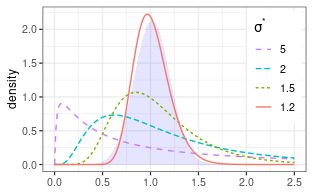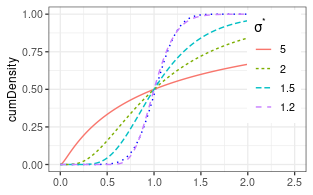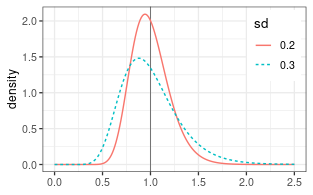# Distribution

## Shape by multiplicative standard deviation

Density distributions of lognormal distributions (lines) get closer to normal density shaded area) as multiplicative standard deviation $$\sigma^*$$ decreases down to 1.2 for same $$\mu^* = 1$$.## Density, distribution function, quantile function and random generation

Are already provided with the base stats package. See ?dlnorm.

## Expected value, Variance, Mode, and Median

getLognormMode(mu = 0.6,sigma = 0.5)
##  1.419068
getLognormMedian(mu = 0.6,sigma = 0.5)
##  1.822119
(theta <- getLognormMoments(mu = 0.6,sigma = 0.5))
##          mean      var        cv
## [1,] 2.064731 1.210833 0.5329404

Mode < Median < Mean for the right-skewed distribution.

The return type of getLognormMoments is a matrix.

# Parameter Estimation from moments

moments <- cbind(mean = c(1,1), var = c(0.2, 0.3)^2 )
(theta <- getParmsLognormForMoments( moments[,1], moments[,2]))
##               mu     sigma
## [1,] -0.01961036 0.1980422
## [2,] -0.04308885 0.2935604The larger the spread, the more skewed is the distribution, here both with an expected value of one.